Colligative Properties : What are the basics of Colligative Properties and its Types

Like pure solids, liquids, and gasses, solutions have well-defined physical properties (e.g., vapor pressure, boiling point, etc.). A change in a property of a solvent that depends on the concentration of dissolved solute(s) particles is called a colligative properties.

Colligative properties do not depend on the identity of either the solvent or the solute(s) particles (kind, size or charge) in the solution.Introduction of Colligative Properties

Colligative properties mean Properties of solutions which depend on the number of solute particles but not on their nature.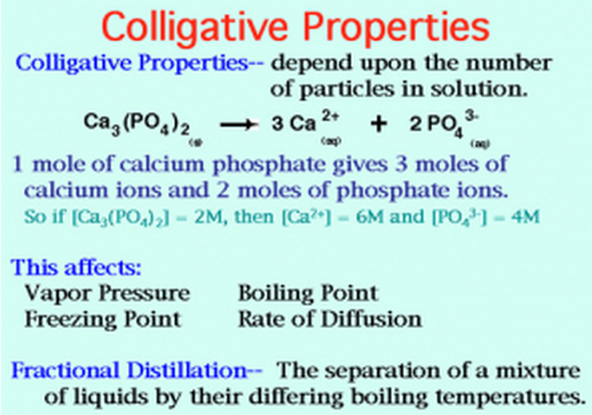Examples of Colligative properties are:

1. Vapour Pressure lowering of a solution
2. Boiling Point Elevation
3. Freezing Point Depression
4. Osmotic Pressure
5. Electrolyte solution
Colligative Properties Example 1:

Vapour Pressure lowering of a solution

The commonality in these properties is that the effects are entropy effects. Take, for example, the vapor pressure of a pure liquid versus one in which a solute has been dissolved. In the former case, the difference in entropy for the phase-change reaction is greater than that for the latter since the process of dissolving the solute into the liquid has slightly increased the entropy of the liquid (more random since the solute is spacing out the solvent molecules a bit). Hence, the vapor pressure of the pure liquid is higher than that of the solution.

Psolvent = csolventsolvent

where Psolvent = vapour pressure of the solvent in solution,

csolvent = mole fraction of the solvent

= # moles of solvent molecules
Total number of moles

andsolvent = vapor pressure of the pure solvent.

Recall that the total pressure of a solution is the sum of the partial pressures of the solvent and solute

psolution = psolvent + psolute = csolventsolvent + csolutesolute

If the solute is non-volatile (no vapor pressure: P°solute = 0) then the total vapor pressure of solution is

psolution = psolvent = csolventsolvent

To see this in graphical form, see the phase diagram for pure water, below. Three lines are present indicating the phase transition (read equilibrium) between solid, liquid and gas. Where all three lines meet is the triple point where all three phases are in equilibrium. We see that at an external pressure of 1 atm. The normal melting point and normal boiling point are indicated. If the pressure is lowered, we note that the boiling point will be lowered and the melting point raised (very slightly; it’s exaggerated here).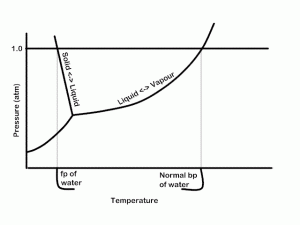Now, look at the following diagram indicating the phase transitions for pure water and for water with some solute dissolved in it (not to scale).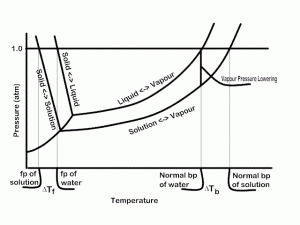Here we see that the curves for equilibrium between the liquid and the other phases are lowered. Look, for example at the vertical line indicating the normal boiling point of water crosses the solution’s lower pressure, i.e., the vapor pressure at that (and any other) temperature is lower for the solution. One could, in principle, calculate the concentration of solute which would be required to create a given VP lowering.

Colligative Properties Example 2:

Boiling Point Elevation

We’ve already seen that in a solution, the vapor pressure of the solution is lowered. Thus, it is reasonable to conclude that one would have to raise the temperature of solution more to bring it to the boiling point.

This point is well illustrated in the Phase diagram for water and solution figured above.

There is a definite relationship between the concentration of solute and the increase in boiling point c.f. the pure liquid.DT = kb m

m = molality of solute = # moles solute / 1000 g solvent

kb = boiling point elevation constant for the liquid. We can use boiling point elevation measurements to determine the molar mass of an unknown solute.

Example:

A sample of 1.20 g of a non-volatile organic compound is dissolved in 60.0 g benzene. The BP of the solution is 80.96°C. BP of pure benzene is 80.08°C. What is the molar mass of the solute?

DT = 80.96 – 80.08 = 0.88°C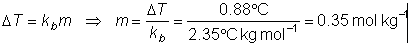but, we only have 60.0 g benzene, not 1000 g. so # moles solute = molality × #kg solvent

NOTE: this is only an approximate molar mass, due to the inaccuracy of the measurement (small temperature effect, hard to measure accurately).

Colligative Properties Example 3:

Freezing Point depression

At the freezing point, solid and liquid are at equilibrium. The temperature where the equilibrium occurs at a pressure of 1.0 atm is called the normal freezing point. The same entropy effects which cause the boiling point to be elevated in a solution cause the freezing point to be depressed. See the figure above for a visual representation of this.

Since at the freezing point, the solid and liquid have the same vapor pressure and since the vapor pressure is lower in the solution than in the pure liquid, it requires a lower temperature to achieve equilibrium. Actually, the solid’s vapor pressure to is affected by solute particles but to a much smaller extent than that of the liquid so we can ignore it for this hand-waving discussion.

The expression for the freezing point depression DTf is:

DTf = kf m

Where kf is the freezing point depression constant for the liquid and m is the molality of the solute.

Example 1:

A solution of 2.95 g of in 100 g cyclohexane had a freezing point of 4.18°C, pure cyclohexane has a FP of 6.50°C. What is the molecular formula of?

DTf = 6.5 – 4.18 = 2.32°C
kf = 20.2°C kg mol-1 (look up in tables)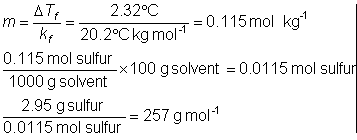This is only the approximate molar mass of the since this technique is not very accurate (only 2 or three sig figs in this experiment).

atomic molar mass of is 32.06 g/mol. It takes 8 atoms of to add up to about 257 g/mol. Thus, the molecular formula for is S8 and the true molar mass is 8 × 32.06 = 256.48 g/mol.

Example 2.

How much glycol (1,2-ethanediol), C2H6O2 must be added to 1.00 L of water so the solution does not freeze above -20°C?

kf (H2O) = 1.86°C kg mol-1
DTf = kf m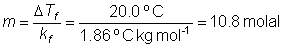since 1.0 L has a mass of 1.0 kg we need 10.8 mol of ethylene glycol

10.8 mol × 62.1 g/mol = 670 g ethylene glycol.

Colligative Properties Example 4:

Osmosis, Osmotic Pressure

Osmosis is a process whereby liquids pass through semi-permeable membranes, spontaneously. This process is driven by changes in entropy. Consider two containers of liquid, connected by a semi-permeable membrane. The semi-permeable membrane can be simply a device which holes small enough to allow the solvent to pass through but not the solute.

In one container is a pure solvent and in the other is the same solvent but with some solute dissolved in it. In this case, there will be solvent passing through in both directions randomly. The rate of the two processes depends on the relative entropy of the two sides and on the relative pressure applied to the solutions.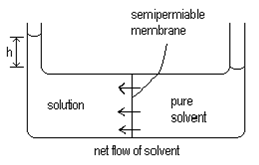Since the solution will have a higher entropy than the solute, it is reasonable to expect that the spontaneous process is the one where the solvent passes through the membrane from the pure solvent side to the solution side where the entropy is higher.

This will occur until the difference in the height of the two columns of liquid (h) is large enough that the pressure caused by this column of liquid exactly stops the net flow of solvent. This pressure is equal to the osmotic pressure.

Osmotic pressure is given the symbol P (Greek equivalent of P) and the equation relating the osmotic pressure to the amount of solute is:

PV = nRT

Note that n/V is concentration in mol/liter so we can also write

P = Csolute RT

P can be measured at room temperature and is, therefore, more useful for measuring solutes which might decompose at higher (boiling point elevation) temperature measurements. It is also extremely sensitive to small amounts of solute and is, therefore, useful for measuring very large molar mass values.

Colligative Properties Example 5:

Electrolyte Solution

IF we dissolve NaCl in H2O we get two ‘particles’ in solution for every one unit of NaCl. Since the Colligative properties depend only on the amount of solute, not on its nature, the concentration of the ‘stuff’ in solution is actually twice that of the nominal concentration of the salt. This must be accounted for in the case of electrolytes dissolving in water. We can use this idea to determine the amount of dissociation of weak electrolytes, we can determine the number of particles a given electrolyte breaks up into. If we assign the symbol i to be the number of ions an electrolyte breaks up into we can rewrite the colligative properties as follows:

DTb = i kb m

DTf = i kfm

PV = inRT

1.